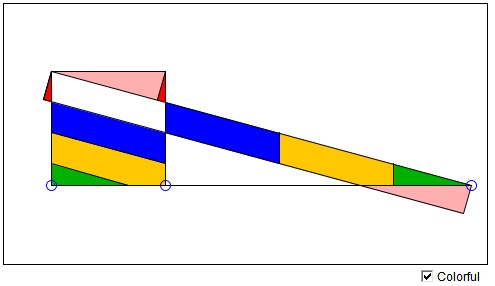# Equidecomposition of a Rectangle and a Square:What is this about? A Mathematical Droodle

### If you are reading this, your browser is not set to run Java applets. Try IE11 or Safari and declare the site https://www.cut-the-knot.org as trusted in the Java setup.What if applet does not run?

ExplanationThe applet purports to illustrate a simple fact:

A rectangle can be cut into several pieces which, after translation and rotation, combine into a square of equal area.

The applet only demonstrates this fact for sufficiently elongated rectangle. Rectangles that are nearer a square to start with can be cut into strips that combine into rectangles of the sort the applet handles well, i.e., in which the length is at least twice its width.Since every triangle is equidecomposable with a rectangle, we can also claim that every triangle is equidecomposable with a square! An even more obvious conclusion is that two rectangles of equal area are equidecomposable. This fact can be established directly and likely in a more economical manner.

(The problem of constructing a square equal in area to a given rectangle has been solved by Euclid's Elements II.14.)

### References

1. G. Frederickson, Dissections: Plane & Fancy, Cambridge University Press, 1997, p. 222### Equidecomposition by Dissiection